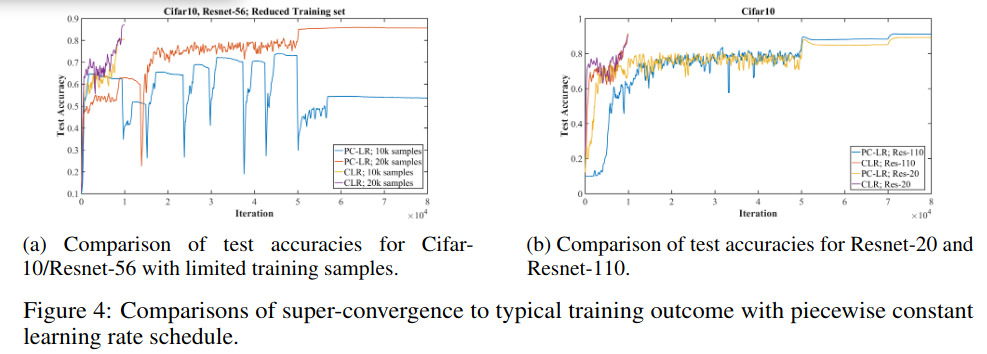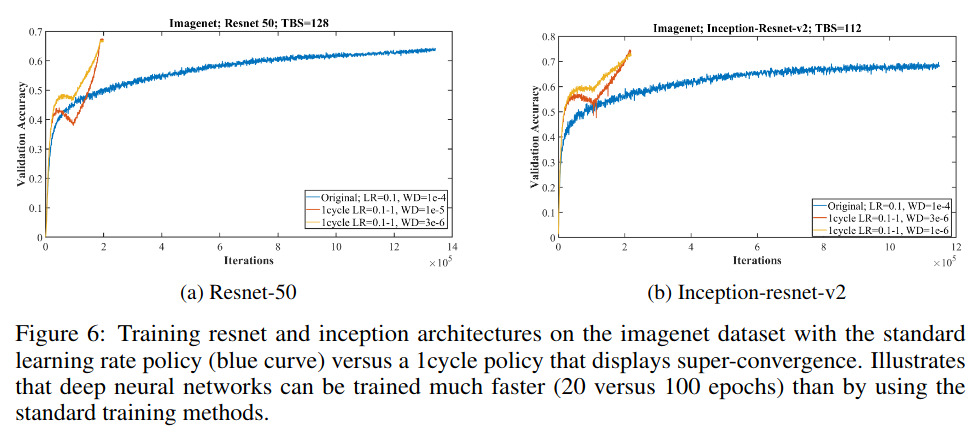# Highlights

The paper behind the pytorch OneCycleLR learning rate scheduler

# Introduction

The goal here is to adjust the learning rate $$\epsilon$$ of a simple stochastic gradient descent: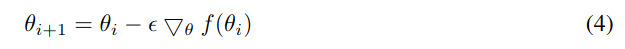They start with the AdaSecant method by Gulcehre et al. which builds an adaptive learning rate method based on the finite difference approximation of the Hessian matrix (denominator):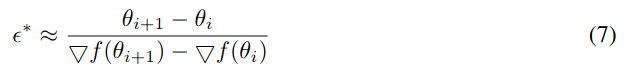Then, by combining Eq.(4) and (7), they get the following updating rule: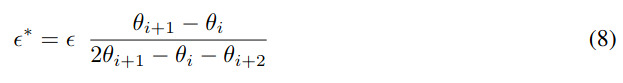The learning rate is then updated with the following moving average: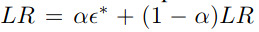Doing so with a fix learning rate at the beginning of the optimization process gives the blue curve in the following figure: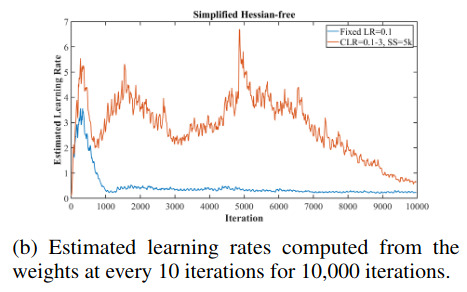However, in their case, they use 2 learning rates : $$LR_{min},LR_{MAX}$$ which they linearly interpolate during a cycle. During the first half of the cycle, the LR goes up from $$LR_{MIN}$$ to $$LR_{MAX}$$ and during the second half the LR goes down.

Doing so leads to the orange curve in the previous plot which corresponds to a larger learning rate than the blue curve and thus a faster convergence.

# Results

Quite amaizing indeed! PC means : piecewise-constant training regime (LR is fixed and then reduced by a factor of X when the validation error plateaus)